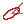Next Previous Up Contents
Next: SkyEllipse Form
Up: Plot Forms
Previous: Error Bars Form

#### A.4.5.7 XYEllipse Form

The XYEllipse form () plots an ellipse (or rectangle, triangle, or other similar figure) defined by two principal radii and an optional angle of rotation, the so-called position angle. This angle, if specified, is in degrees and gives the angle counterclockwise from the horizontal axis to the first principal radius.Example XYEllipse plotXYEllipse form configuration panel

The configuration options are:

See Appendix A.4.6.
The two principal radii for the ellipse, in the same units as the position coordinates. It doesn't matter whether the primary is larger than the secondary. If the Secondary Radius field is left blank, it is assumed to equal the Primary Radius field, i.e. the ellipses are circles.
Orientation
Position angle in degrees. This is the angle anticlockwise from the X axis to the primary radius. If the value is missing (either this field not filled in or blank in the data) it is considered to be zero.
Ellipse
Ellipse graphical representation, selected from a range of options (includes also rectangles, crosses etc).
Scale
Changes the factor by which all ellipse sizes are scaled. If the ellipses are too small, slide it right, if they are too big, slide it left. The slider scale is logarithmic. Alternatively, enter a fixed value in the text field.
Auto Scale
If selected, this option will determine the default ellipse scale size from the data - it will fix it so that the largest ellipses are a few tens of pixels long by default. That scaling can then be adjusted using the Scale slider. If unselected, then the default position of the Scale slider corresponds to the actual positions given by the submitted ellipse radii coordinates.

Next Previous Up Contents
Next: SkyEllipse Form
Up: Plot Forms
Previous: Error Bars Form

TOPCAT - Tool for OPerations on Catalogues And Tables